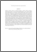# Novel bivariate moment-closure approximations

## Citation

Krishnarajah, Isthrinayagy and Marion, Glenn and Gibson, Gavin (2007) Novel bivariate moment-closure approximations. Mathematical Biosciences, 208 (2). pp. 621-643. ISSN 0025-5564

## Abstract

Nonlinear stochastic models are typically intractable to analytic solutions and hence, moment-closure schemes are used to provide approximations to these models. Existing closure approximations are often unable to describe transient aspects caused by extinction behaviour in a stochastic process. Recent work has tackled this problem in the univariate case. In this study, we address this problem by introducing novel bivariate moment-closure methods based on mixture distributions. Novel closure approximations are developed, based on the beta-binomial, zero-modified distributions and the log-Normal, designed to capture the behaviour of the stochastic SIS model with varying population size, around the threshold between persistence and extinction of disease. The idea of conditional dependence between variables of interest underlies these mixture approximations. In the first approximation, we assume that the distribution of infectives (I) conditional on population size (N) is governed by the beta-binomial and for the second form, we assume that I is governed by zero-modified beta-binomial distribution where in either case N follows a log-Normal distribution. We analyse the impact of coupling and inter-dependency between population variables on the behaviour of the approximations developed. Thus, the approximations are applied in two situations in the case of the SIS model where: (1) the death rate is independent of disease status; and (2) the death rate is disease-dependent. Comparison with simulation shows that these mixture approximations are able to predict disease extinction behaviour and describe transient aspects of the process.Preview
PDF (Abstract)
Novel bivariate moment.pdfView Item